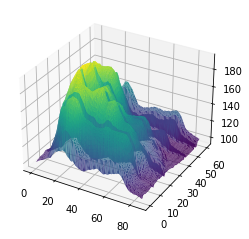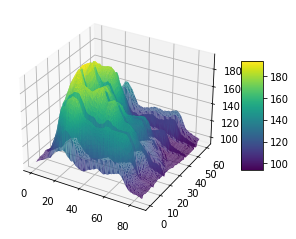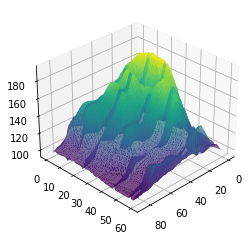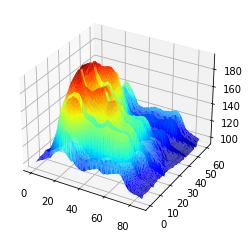3D plots are awesome to make surface plots. In a surface plot, each point is defined by 3 variables: its latitude, its longitude, and its altitude (X, Y and Z). Thus, 2 types of inputs are possible: 1) A rectangular matrix where each cell represents the altitude.
2) A long format matrix with 3 columns where each row is a point.

This example uses the rectangular format as an input, transforms it to a long format, and makes the plot:

``````# libraries
from mpl_toolkits.mplot3d import Axes3D
import matplotlib.pyplot as plt
import pandas as pd
import seaborn as sns

# Get the data (csv file is hosted on the web)
url = 'https://raw.githubusercontent.com/holtzy/The-Python-Graph-Gallery/master/static/data/volcano.csv'

# Transform it to a long format
df=data.unstack().reset_index()
df.columns=["X","Y","Z"]

# And transform the old column name in something numeric
df['X']=pd.Categorical(df['X'])
df['X']=df['X'].cat.codes

# Make the plot
fig = plt.figure()
ax = fig.gca(projection='3d')
ax.plot_trisurf(df['Y'], df['X'], df['Z'], cmap=plt.cm.viridis, linewidth=0.2)
plt.show()

# to Add a color bar which maps values to colors.
fig = plt.figure()
ax = fig.gca(projection='3d')
surf=ax.plot_trisurf(df['Y'], df['X'], df['Z'], cmap=plt.cm.viridis, linewidth=0.2)
fig.colorbar( surf, shrink=0.5, aspect=5)
plt.show()

# Rotate it
fig = plt.figure()
ax = fig.gca(projection='3d')
surf=ax.plot_trisurf(df['Y'], df['X'], df['Z'], cmap=plt.cm.viridis, linewidth=0.2)
ax.view_init(30, 45)
plt.show()

# Other palette
fig = plt.figure()
ax = fig.gca(projection='3d')
ax.plot_trisurf(df['Y'], df['X'], df['Z'], cmap=plt.cm.jet, linewidth=0.01)
plt.show()``````## Contact & Edit

👋 This document is a work by Yan Holtz. You can contribute on github, send me a feedback on twitter or subscribe to the newsletter to know when new examples are published! 🔥